-400
0.25 to 1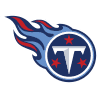Derrick Henry
45.8% implied probability

+900
9 to 1Jonathan Taylor
5.7% implied probability

+1000
10 to 1Ezekiel Elliott
5.2% implied probability

+1400
14 to 1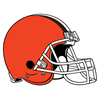Nick Chubb
3.8% implied probability

+1400
14 to 1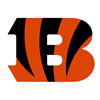Joe Mixon
3.8% implied probability

+2500
25 to 1James Conner
2.2% implied probability

+3500
35 to 1James Robinson
1.6% implied probability

+6500
65 to 1Dalvin Cook
0.9% implied probability

+8000
80 to 1Aaron Jones
0.7% implied probability

+10000
100 to 1Miles Sanders
0.6% implied probability

+10000
100 to 1Alexander Mattison
0.6% implied probability

+10000
100 to 1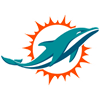Myles Gaskin
0.6% implied probability

+10000
100 to 1Josh Jacobs
0.6% implied probability

+10000
100 to 1Darrell Henderson
0.6% implied probability

+10000
100 to 1Devin Singletary
0.6% implied probability

+10000
100 to 1Sony Michel
0.6% implied probability

+10000
100 to 1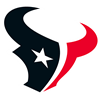Phillip Lindsay
0.6% implied probability

+10000
100 to 1Chase Edmonds
0.6% implied probability

+10000
100 to 1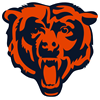David Montgomery
0.6% implied probability

+10000
100 to 1Damien Harris
0.6% implied probability

+10000
100 to 1Tony Pollard
0.6% implied probability

+10000
100 to 1Kyler Murray
0.6% implied probability

+10000
100 to 1Austin Ekeler
0.6% implied probability

+10000
100 to 1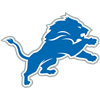D'Andre Swift
0.6% implied probability

+10000
100 to 1Jalen Hurts
0.6% implied probability

+10000
100 to 1Clyde Edwards-Helaire
0.6% implied probability

+10000
100 to 1Zack Moss
0.6% implied probability

+10000
100 to 1Antonio Gibson
0.6% implied probability

+10000
100 to 1Javonte Williams
0.6% implied probability

+10000
100 to 1Trey Sermon
0.6% implied probability

+10000
100 to 1Najee Harris
0.6% implied probability

+10000
100 to 1AJ Dillon
0.6% implied probability

+10000
100 to 1Leonard Fournette
0.6% implied probability

+10000
100 to 1Lamar Jackson
0.6% implied probability

+10000
100 to 1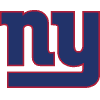Saquon Barkley
0.6% implied probability

+10000
100 to 1Michael Carter
0.6% implied probability

+10000
100 to 1Alvin Kamara
0.6% implied probability

+10000
100 to 1Mike Davis
0.6% implied probability

+10000
100 to 1Kenyan Drake
0.6% implied probability

+10000
100 to 1Kareem Hunt
0.6% implied probability

+10000
100 to 1David Johnson
0.6% implied probability

+10000
100 to 1Latavius Murray
0.6% implied probability

+10000
100 to 1Chris Carson
0.6% implied probability

+10000
100 to 1Damien Williams
0.6% implied probability

+10000
100 to 1Melvin Gordon
0.6% implied probability

+10000
100 to 1Christian McCaffrey
0.6% implied probability

+15000
150 to 1Jamaal Williams
0.4% implied probability

+15000
150 to 1Jeff Wilson
0.4% implied probability

+15000
150 to 1Tevin Coleman
0.4% implied probability

+15000
150 to 1Raheem Mostert
0.4% implied probability

+20000
200 to 1La'Mical Perine
0.3% implied probability

+20000
200 to 1Anthony McFarland
0.3% implied probability

+20000
200 to 1Ronald Jones
0.3% implied probability

+20000
200 to 1Josh Allen
0.3% implied probability

+20000
200 to 1Matt Breida
0.3% implied probability

+20000
200 to 1Mark Ingram
0.3% implied probability

+20000
200 to 1J.D. McKissic
0.3% implied probability

+20000
200 to 1Marlon Mack
0.3% implied probability

+20000
200 to 1Taysom Hill
0.3% implied probability

+20000
200 to 1Darrel Williams
0.3% implied probability

+25000
250 to 1Kenneth Gainwell
0.2% implied probability

+25000
250 to 1Russell Wilson
0.2% implied probability

+25000
250 to 1Larry Rountree
0.2% implied probability

+25000
250 to 1Justin Fields
0.2% implied probability

+25000
250 to 1Chuba Hubbard
0.2% implied probability

+25000
250 to 1Trey Lance
0.2% implied probability

+25000
250 to 1Justin Jackson
0.2% implied probability

+25000
250 to 1Malcolm Brown
0.2% implied probability

+25000
250 to 1Daniel Jones
0.2% implied probability

+25000
250 to 1Peyton Barber
0.2% implied probability

+50000
500 to 1Carlos Hyde
0.1% implied probability

+50000
500 to 1Ryan Tannehill
0.1% implied probability

+50000
500 to 1Devontae Booker
0.1% implied probability

+50000
500 to 1Kerryon Johnson
0.1% implied probability

+50000
500 to 1Nyheim Hines
0.1% implied probability

+50000
500 to 1Giovani Bernard
0.1% implied probability

+50000
500 to 1Patrick Mahomes
0.1% implied probability

+50000
500 to 1Benny Snell
0.1% implied probability

+50000
500 to 1Carson Wentz
0.1% implied probability

+50000
500 to 1Justin Herbert
0.1% implied probability

+50000
500 to 1Salvon Ahmed
0.1% implied probability

+50000
500 to 1Zach Wilson
0.1% implied probability

+50000
500 to 1James White
0.1% implied probability

+50000
500 to 1Jermar Jefferson
0.1% implied probability

+50000
500 to 1Darrynton Evans
0.1% implied probability

+50000
500 to 1Sam Darnold
0.1% implied probability

+50000
500 to 1Dak Prescott
0.1% implied probability

+50000
500 to 1Tarik Cohen
0.1% implied probability

+50000
500 to 1Tyrod Taylor
0.1% implied probability

+50000
500 to 1Boston Scott
0.1% implied probability

+50000
500 to 1Tua Tagovailoa
0.1% implied probability

+50000
500 to 1Joe Burrow
0.1% implied probability

+50000
500 to 1Trevor Lawrence
0.1% implied probability